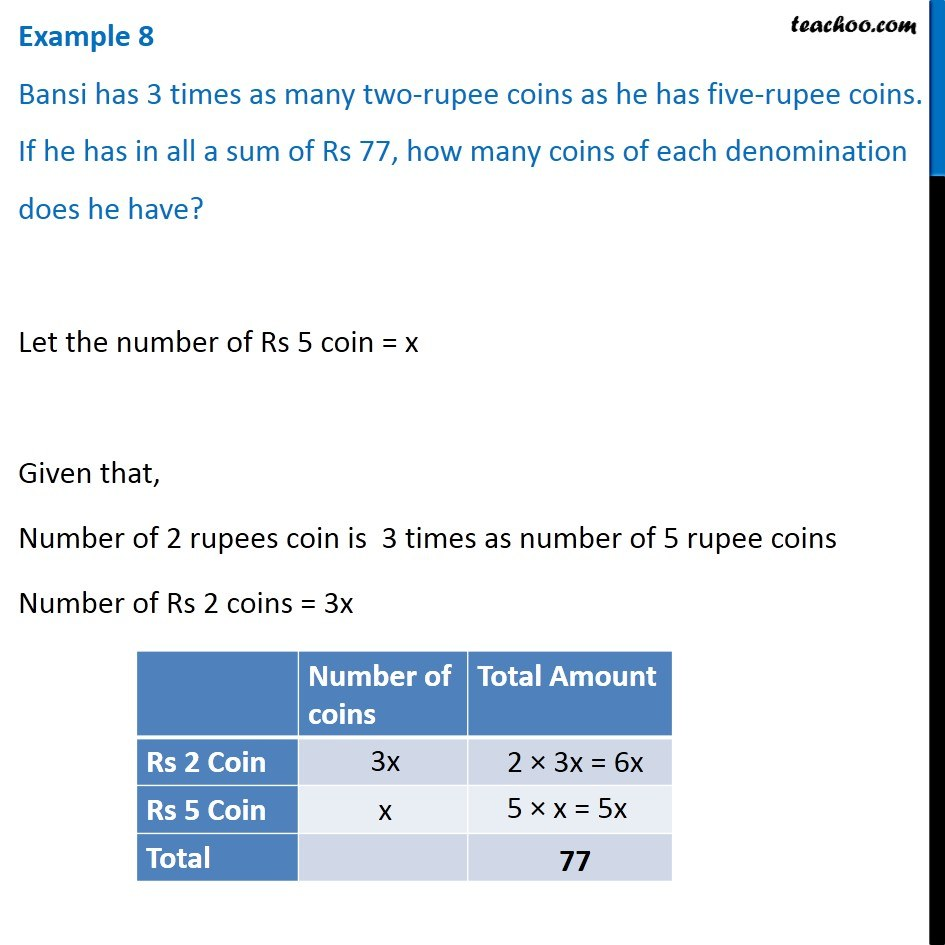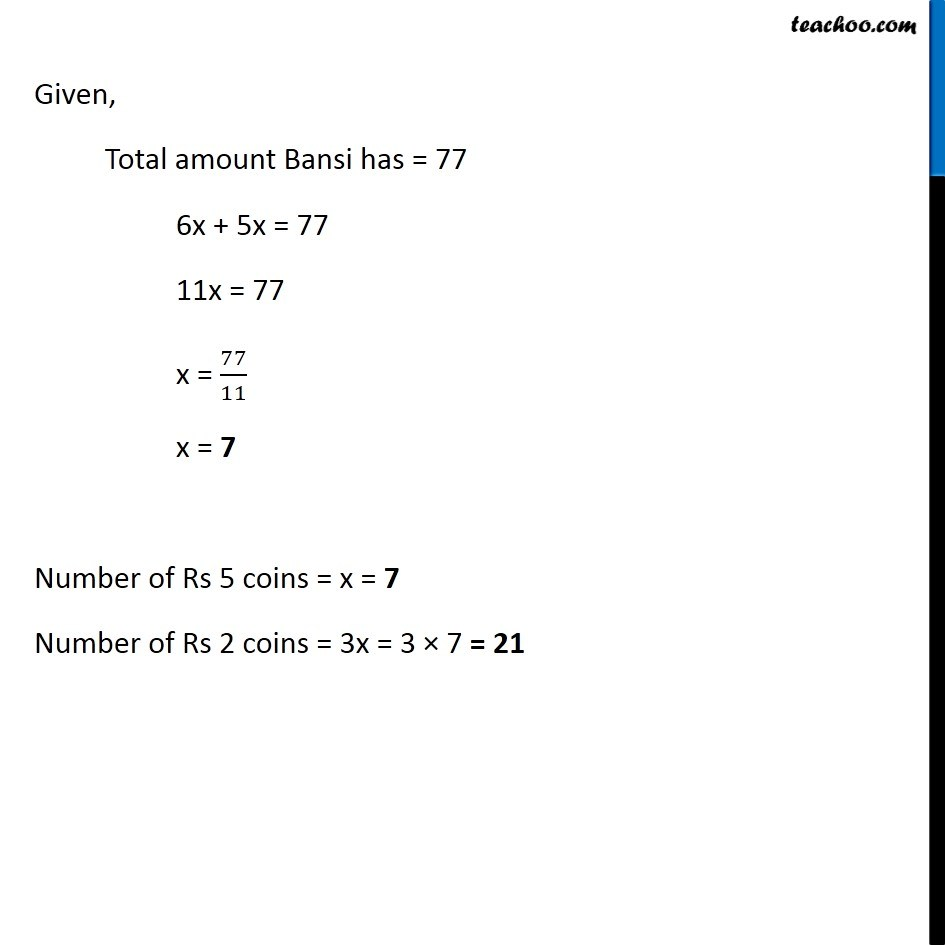1. Chapter 2 Class 8 Linear Equations in One Variable
2. Concept wise
3. Forming Linear Equations - Coins and Currency Notes

Transcript

Example 8 Bansi has 3 times as many two-rupee coins as he has five-rupee coins. If he has in all a sum of Rs 77, how many coins of each denomination does he have?Let the number of Rs 5 coin = x Given that, Number of 2 rupees coin is 3 times as number of 5 rupee coins Number of Rs 2 coins = 3x Given, Total amount Bansi has = 77 6x + 5x = 77 11x = 77 x = 77/11 x = 7 Number of Rs 5 coins = x = 7 Number of Rs 2 coins = 3x = 3 × 7 = 21